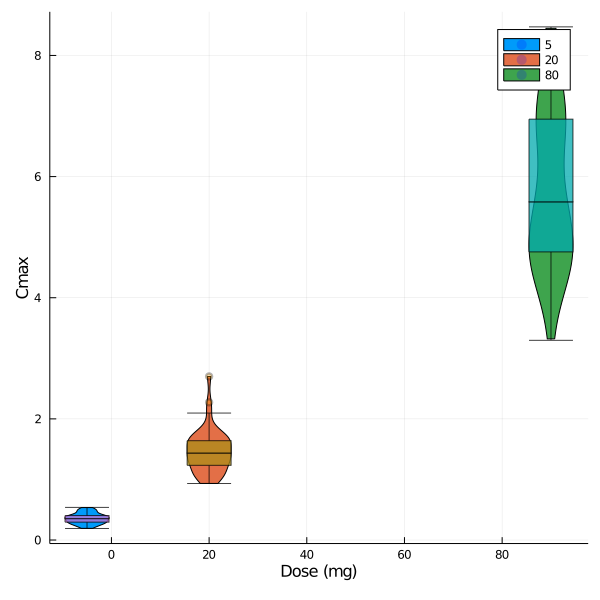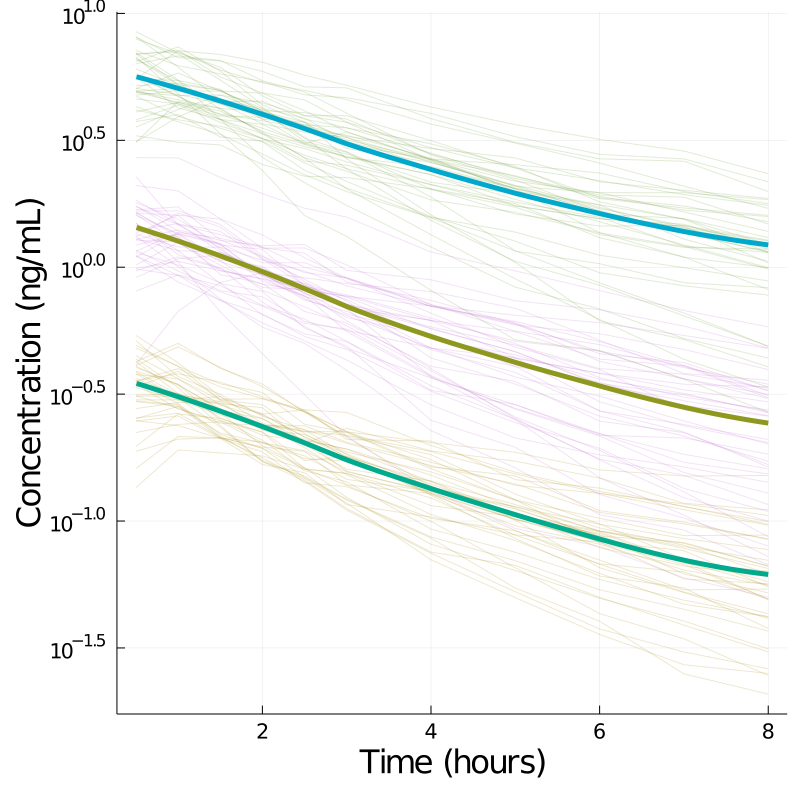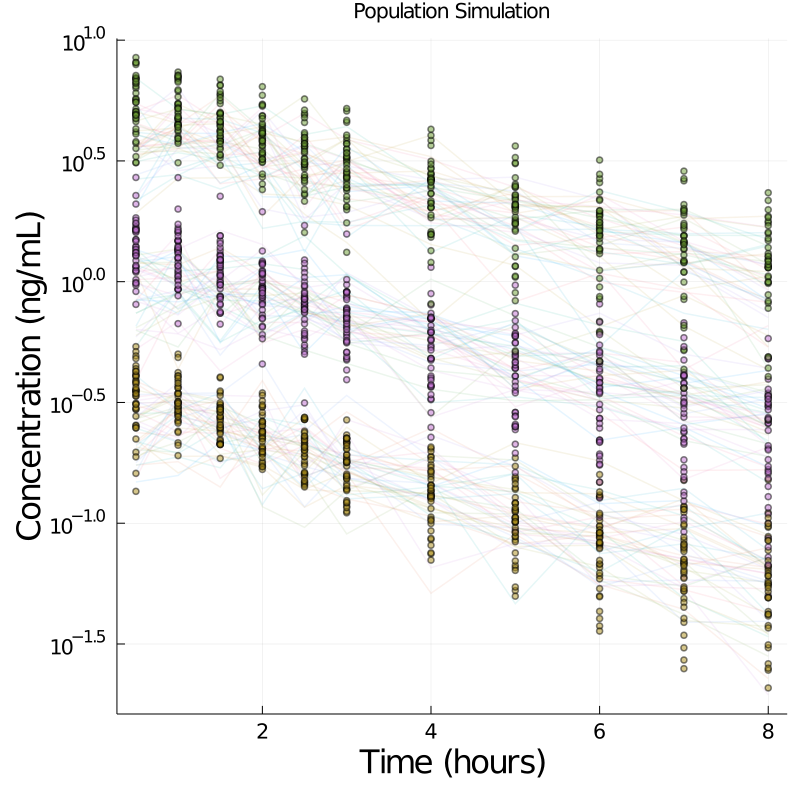# Introduction

This tutorial provides a comprehensive introduction to a modeling and simulation workflow in Pumas. This tutorial will not get into the details of Pumas specifics, but instead provide a narrative on the lines of a regular workflow in our day to day work, with brevity where required to allow a broad overview. Wherever possible, cross-references will be provided to documentation and detailed examples that provide deeper insght into a particular topic.

As part of this workflow you will be introduced to various aspects such as

1. Data wrangling in Julia

2. Exploratory analysis in Julia

3. Continous and discrete data non-linear mixed effects modeling in Pumas

4. Model comparison routines, post-processing, validation etc.

## The study and design

CTMNopain is a novel anti-inflammatory agent under preliminary investigation. A dose-ranging trial was conducted comparing placebo with 3 doses of CTMNopain (5mg, 20mg and 80 mg QD). The maximum tolerated dose is 160 mg per day. Plasma concentrations (mg/L) of the drug were measured at 0, 0.5, 1, 1.5, 2, 2.5, 3-8 hours.

Pain score (0=no pain, 1=mild, 2=moderate, 3=severe) were obtained at time points when plasma concentration was collected. A pain score of 2 or more is considered as no pain relief.

The subjects can request for remedication if pain relief is not achieved after 2 hours post dose. Some subjects had remedication before 2 hours if they were not able to bear the pain. The time to remedication and the remedication status is available for subjects.

## The goal

We are expected to provide input for an optimal dose/dosing regimen to be carried forward in future trials. Two datasets are provided below, that can be downloaded using the links below.

using PumasTutorials
using Random
using CSV
using Pumas
using PumasPlots
using Plots
using StatsPlots
using Pipe
using StatsBase
using PrettyTables


### Data Wrangling

We start by reading in the two dataset and making some quick summaries.

Note: As a general convention during this example, I will refer to dataframes by ending the name of the variable with df and the Population version of that dataframe will be without the df to avoid confusion.

pkpain_df = DataFrame(CSV.File(joinpath(dirname(pathof(PumasTutorials)), "..", "data", "intro", "pk_painscore.csv"), missingstrings=["", "NA", "."]))
remed_df  = DataFrame(CSV.File(joinpath(dirname(pathof(PumasTutorials)), "..", "data", "intro", "pain_remedication.csv"), missingstrings=["", "NA", "."]))


Let's filter out the placebo data as we don't need that for the PK analysis.

pkpain_noplb_df = filter(x -> !(occursin.("Placebo", x.ARM)), pkpain_df)
pretty_table(first(pkpain_noplb_df,10), backend = :html;
formatters = ft_round(3))

ARM ID TIME CONC PAINRELIEF DOSE
String Int64 Float64 Float64 Int64 Int64
A20_0_at2h 1.0 0.0 0.0 0.0 20.0
A20_0_at2h 1.0 0.5 1.156 1.0 20.0
A20_0_at2h 1.0 1.0 1.372 1.0 20.0
A20_0_at2h 1.0 1.5 1.301 1.0 20.0
A20_0_at2h 1.0 2.0 1.192 1.0 20.0
A20_0_at2h 1.0 2.5 1.136 1.0 20.0
A20_0_at2h 1.0 3.0 0.873 1.0 20.0
A20_0_at2h 1.0 4.0 0.74 1.0 20.0
A20_0_at2h 1.0 5.0 0.6 0.0 20.0
A20_0_at2h 1.0 6.0 0.426 1.0 20.0

do some data wrangling and plotting here

### Non-compartmental analysis

Let's begin by peforming a quick NCA of the concentration time profiles and view the exposure changes across doses. The input data specicfication for NCA analysis () requires the presence of a route column and an amount column that specifies the dose. So, let's add that in.

#adding route variable
pkpain_noplb_df[!,:route] .= "ev"
# creating an amt column
pkpain_noplb_df[!,:amt] .= ifelse.(pkpain_noplb_df.TIME .== 0, pkpain_noplb_df.DOSE, missing)


Now, we map the data variables to the read_nca function that prepares the data for NCA analysis.

pkpain_nca = read_nca(pkpain_noplb_df,
id = :ID,
time = :TIME,
amt = :amt,
conc = :CONC,
group = [:DOSE],
route = :route)


A full NCAReport is now obtained for completeness purposes, but later we will only extract a couple of key metrics of interest.

pk_nca_report = NCAReport(pkpain_nca, sigdig=3)
pretty_table(first(pk_nca_report,10), backend = :html;
formatters = ft_round(3))

id DOSE doseamt tlag tmax cmax tlast clast clast_pred auclast kel half_life aucinf_obs aucinf_pred vz_f_obs cl_f_obs vz_f_pred cl_f_pred n_samples cmax_dn auclast_dn aucinf_dn_obs auc_extrap_obs aucinf_dn_pred auc_extrap_pred aumclast aumcinf_obs aumc_extrap_obs aumcinf_pred aumc_extrap_pred n_samples_kel rsq_kel rsq_adj_kel corr_kel intercept_kel kel_t_low kel_t_high span route run_status
Int64 Int64 Int64 Float64 Float64 Float64 Float64 Float64 Float64 Float64 Float64 Float64 Float64 Float64 Float64 Float64 Float64 Float64 Int64 Float64 Float64 Float64 Float64 Float64 Float64 Float64 Float64 Float64 Float64 Float64 Int64 Float64 Float64 Float64 Float64 Float64 Float64 Float64 String Symbol
6.0 5.0 5.0 0.0 1.0 0.316 8.0 0.111 0.107 1.557 0.151 4.595 2.29 2.266 14.474 2.184 14.624 2.206 12.0 0.063 0.311 0.458 32.022 0.453 31.318 5.276 16.002 67.028 15.659 66.306 6.0 0.983 0.979 0.991 -1.027 3.0 8.0 1.088 EV Success
8.0 5.0 5.0 0.0 0.5 0.336 8.0 0.096 0.096 1.498 0.145 4.768 2.16 2.159 15.922 2.315 15.93 2.316 12.0 0.067 0.3 0.432 30.642 0.432 30.61 4.93 14.778 66.639 14.763 66.605 3.0 1.0 0.999 1.0 -1.18 6.0 8.0 0.419 EV Success
9.0 5.0 5.0 0.0 0.5 0.539 8.0 0.043 0.039 1.37 0.311 2.226 1.508 1.494 10.648 3.316 10.749 3.347 12.0 0.108 0.274 0.302 9.175 0.299 8.32 3.431 4.982 31.135 4.824 28.884 9.0 0.993 0.992 0.997 -0.761 1.5 8.0 2.92 EV Success
12.0 5.0 5.0 0.0 1.0 0.215 8.0 0.066 0.065 1.063 0.184 3.759 1.424 1.418 19.039 3.511 19.125 3.527 12.0 0.043 0.213 0.285 25.32 0.284 24.981 3.584 8.424 57.453 8.337 57.012 9.0 0.991 0.989 0.995 -1.253 1.5 8.0 1.729 EV Success
17.0 5.0 5.0 0.0 1.0 0.19 8.0 0.056 0.055 0.948 0.242 2.863 1.179 1.177 17.517 4.241 17.552 4.25 12.0 0.038 0.19 0.236 19.579 0.235 19.416 3.226 6.025 46.466 5.997 46.209 3.0 0.995 0.989 0.997 -0.958 6.0 8.0 0.699 EV Success
24.0 5.0 5.0 0.0 1.0 0.264 8.0 0.025 0.024 0.908 0.349 1.986 0.979 0.976 14.634 5.109 14.677 5.124 12.0 0.053 0.182 0.196 7.211 0.195 6.941 2.431 3.198 23.978 3.167 23.236 7.0 0.995 0.994 0.998 -0.952 2.5 8.0 2.77 EV Success
29.0 5.0 5.0 0.0 0.5 0.353 8.0 0.038 0.037 1.171 0.308 2.248 1.293 1.29 12.54 3.867 12.571 3.877 12.0 0.071 0.234 0.259 9.451 0.258 9.225 3.179 4.553 30.177 4.516 29.616 10.0 0.992 0.991 0.996 -0.838 1.0 8.0 3.114 EV Success
32.0 5.0 5.0 0.0 0.5 0.311 8.0 0.151 0.143 1.592 0.084 8.287 3.399 3.305 17.584 1.471 18.086 1.513 12.0 0.062 0.318 0.68 53.17 0.661 51.834 5.784 41.853 86.181 39.971 85.53 9.0 0.968 0.963 0.984 -1.274 1.5 8.0 0.784 EV Success
38.0 5.0 5.0 0.0 0.5 0.295 8.0 0.077 0.076 1.168 0.132 5.235 1.751 1.741 21.57 2.856 21.689 2.872 12.0 0.059 0.234 0.35 33.286 0.348 32.916 3.721 12.784 70.89 12.634 70.544 6.0 0.996 0.995 0.998 -1.519 3.0 8.0 0.955 EV Success
40.0 5.0 5.0 0.0 0.5 0.449 8.0 0.065 0.065 1.384 0.185 3.738 1.734 1.732 15.546 2.883 15.567 2.887 12.0 0.09 0.277 0.347 20.214 0.346 20.108 3.93 8.625 54.434 8.594 54.271 5.0 0.998 0.997 0.999 -1.256 4.0 8.0 1.07 EV Success

As CTMNopain's effect maybe mainly related to maximum concentration (cmax) or area under the curve (auc), we present some summary statistics.

auc_cmax = select(pk_nca_report, [:id, :DOSE, :cmax, :aucinf_obs])
#pivot data to allow easy computation
auc_cmax_stacked = stack(auc_cmax, [:cmax, :aucinf_obs], [:id, :DOSE])
auc_cmax_summary = combine(groupby(auc_cmax_stacked,[:DOSE,:variable]),
[col => fun for col in [:value]
for fun in [mean, median, geomean, std]])

pretty_table(auc_cmax_summary, backend = :html;
formatters = ft_round(3, [3,4,5,6]))

DOSE variable value_mean value_median value_geomean value_std
Int64 CategoricalArrays.CategoricalValue{String,UInt32} Float64 Float64 Float64 Float64
5 cmax 0.356 0.353 0.345 0.088
20 cmax 1.474 1.436 1.434 0.362
80 cmax 5.787 5.583 5.641 1.32
5 aucinf_obs 1.598 1.532 1.534 0.49
20 aucinf_obs 6.376 6.459 6.02 2.223
80 aucinf_obs 29.502 28.016 28.297 8.696
#TODO unable to get ordered x-axis
auc_cmax[!,:Dose] .= CategoricalArray(auc_cmax.DOSE)
#levels!(auc_cmax[!,:Dose], ["5", "20", "80"])
@df auc_cmax groupedviolin(:DOSE, :cmax, group=:Dose,
marker=(0.2,:blue,stroke(0)))
@df auc_cmax groupedboxplot!(:DOSE, :cmax , group=:Dose, label="",
marker=(0.3,:orange,stroke(2)), alpha=0.75)
plot!(size = (600,600), xlabel = "Dose (mg)", ylabel = "Cmax")Dose normalized PK parameters, cmax and aucinf were essentially dose proportional between for 5 mg, 20 mg and 80 mg doses. Based on visual inspection of the concentration time profiles as seen below, CTMNopain exhibited monophasic decline, and perhaps a one compartment model best fits the PK data.

pkpain_noplb_plot_df = filter(x -> !(x.TIME .== 0), pkpain_noplb_df)
@df pkpain_noplb_plot_df plot(:TIME, :CONC,
color=:DOSE, group=:ID, legend=false,
yaxis =:log, alpha=0.2)

@df pkpain_noplb_plot_df plot!(:TIME, :CONC,
group=:DOSE, legend=false, linewidth = 5,
yaxis =:log, seriestype = :Loess,
xlabel = "Time (hours)", ylabel = "Concentration (ng/mL)",
size = (800, 800), guidefontsize = 22,
tickfontsize =14)### Pharmacokinetic modeling

As seen from the plot above, the concentrations decline monoexponentially. We will evaluate both one and two compartment structural models to assess best fit. Further, different residual error models will also be tested.

We will use the results from NCA to provide us good initial estimates. The mean clearance is 3.29, the mean volume is 16.45 and a good initial estimate for absorption rate as obtained by $0.693/(tmax/4)$ is 3.85

#### Data preparation for modeling

PumasNDF requires the presence of evid and cmt columns in the dataset.

pkpain_noplb_df[!, :evid] .= ifelse.(pkpain_noplb_df.TIME .== 0, 1, 0)
pkpain_noplb_df[!, :cmt] .= ifelse.(pkpain_noplb_df.TIME .== 0, 1, 2)
pkpain_noplb_df[!, :cmt2] .= 1 # for zero order absorption


Further, observations at time of dosing, i.e., when evid = 1 have to be missing

pkpain_noplb_df[!, :CONC] .= ifelse.(pkpain_noplb_df.evid .== 1, missing, pkpain_noplb_df.CONC)


The dataframe is now converted to a Population using read_pumas. Note that both observations and covariates are required to be an array even if it is one element.

pkpain_noplb = read_pumas(pkpain_noplb_df,
id           = :ID,
time         = :TIME,
amt          = :amt,
observations = [:CONC],
covariates   = [:DOSE],
evid         = :evid,
cmt          = :cmt)


Now that the data is transformed to a Population of subjects, we can explore different models.

#### One-compartment model

pk_1cmp = @model begin
@param begin
tvcl ∈ RealDomain(lower = 0, init = 3.2)
tvv  ∈ RealDomain(lower = 0, init = 16.4)
tvka ∈ RealDomain(lower = 0, init = 3.8)
Ω    ∈ PDiagDomain(init = [0.04,0.04,0.04])
σ_p  ∈ RealDomain(lower = 0.0001, init = 0.2)
end
@random begin
η ~ MvNormal(Ω)
end
@covariates DOSE
@pre begin
CL = tvcl * exp(η)
Vc = tvv * exp(η)
Ka = tvka * exp(η)
end
@dynamics Depots1Central1
@derived begin
cp := @. Central/Vc
CONC ~ @. Normal(cp, cp*σ_p)
end
end

PumasModel
Parameters: tvcl, tvv, tvka, Ω, σ_p
Random effects: η
Covariates: DOSE
Dynamical variables: Depot, Central
Derived: CONC
Observed: CONC


Before going to fit the model, let's evaluate some helpful steps.

1. Simulation to check appropriatness of data and model

simpk = simobs(pk_1cmp, pkpain_noplb, init_param(pk_1cmp))
plot(simpk, xlabel = "Time (hours)", ylabel = "Concentration (ng/mL)",
size = (800, 800), guidefontsize = 22, label = "Simulated profiles",
tickfontsize =14, alpha=0.1, yaxis = :log)
#
@df pkpain_noplb_plot_df scatter!(:TIME, :CONC,
color=:DOSE, group=:ID, legend=false,
alpha=0.5, yaxis = :log, label = "Observed concentrations")Our NCA based initial guess on the parameters seem to work well.

Lets change the initial estimate of a couple of the parameters to evaluate the senstitivty.

pkparam = (init_param(pk_1cmp)..., tvka=2, tvv = 10)

(tvcl = 3.2, tvv = 10, tvka = 2, Ω = PDMats.PDiagMat{Float64,Array{Float64,
1}}(3, [0.04, 0.04, 0.04], [25.0, 25.0, 25.0]), σ_p = 0.2)

simpk = simobs(pk_1cmp,
pkpain_noplb,
pkparam)
plot(simpk, xlabel = "Time (hours)", ylabel = "Concentration (ng/mL)",
size = (800, 800), guidefontsize = 22,
tickfontsize =14, alpha=0.1, yaxis = :log)
#
@df pkpain_noplb_plot_df scatter!(:TIME, :CONC,
color=:DOSE, group=:ID, legend=false,
alpha=0.5, yaxis = :log)